# Double of a Riemann surface

(diff) ← Older revision | Latest revision (diff) | Newer revision → (diff)
A two-sheeted covering surfaceof a finite Riemann surface. Each interior point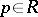is brought into correspondence with a pair of pointsandof the double; in other words, two conjugate pointsand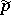are situated over. Each pointof the boundary ofis brought into correspondence with a point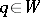. Moreover, two non-intersecting neighbourhoods of the points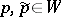are situated over each neighbourhood of an interior point. Ifis a local uniformizing parameter in a neighbourhood of the interior point, it will also be a local uniformizing parameter in a-neighbourhood of one out of the two conjugate points oflying over, say in a-neighbourhood of the point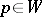; then, in a-neighbourhood of the conjugate point, the complex conjugateof the variablewill be a local uniformizing parameter. Ifis a local uniformizing parameter at a pointof the boundary of, then the variable which is equal toon one sheet ofand to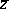on the other will be a local uniformizing parameter at the pointlying over it.
In the case of a compact orientable Riemann surface, the double simply consists of two compact orientable Riemann surfaces, and its study is accordingly of no interest. In all other cases the double of the Riemann surface is a compact orientable Riemann surface. This fact permits one to simplify the study of certain problems in the theory of functions onby reducing them to the study of functions on. The genus (cf. Genus of a surface) ofis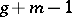, whereis the genus ofandis the number of components of the boundary of, which are assumed to be non-degenerate. For instance, the double of a simply-connected plane domain is a sphere, while the double of an-connected plane domain is a sphere withhandles.
Analytic differentials on a Riemann surface (cf. Differential on a Riemann surface)are analytic differentials on the doublecharacterized by the fact that they assume conjugate values at conjugate points ofand take real values at the pointslying over points of the boundary of.